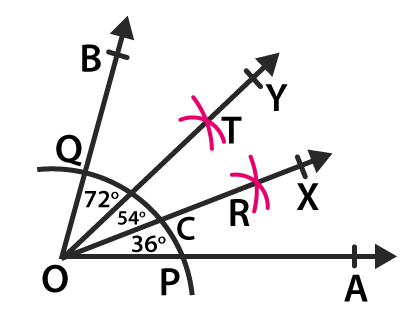# RD Sharma Solutions for Class 6 Maths Chapter 19: Geometrical Constructions Exercise 19.5

This exercise contains problems which help students understand the construction of angles based on given conditions. The concepts are explained in an interactive manner to improve grasping capacity among students. The solutions are made available in PDF format so that the students can download exercise-wise answers to speed up their exam preparation. For a better understanding of the concepts which are explained in this exercise, students can avail RD Sharma Solutions Class 6 Maths Chapter 19 Geometrical Constructions Exercise 19.5 PDF from the links which are available here.

## RD Sharma Solutions for Class 6 Maths Chapter 19: Geometrical Constructions Exercise 19.5 Download PDF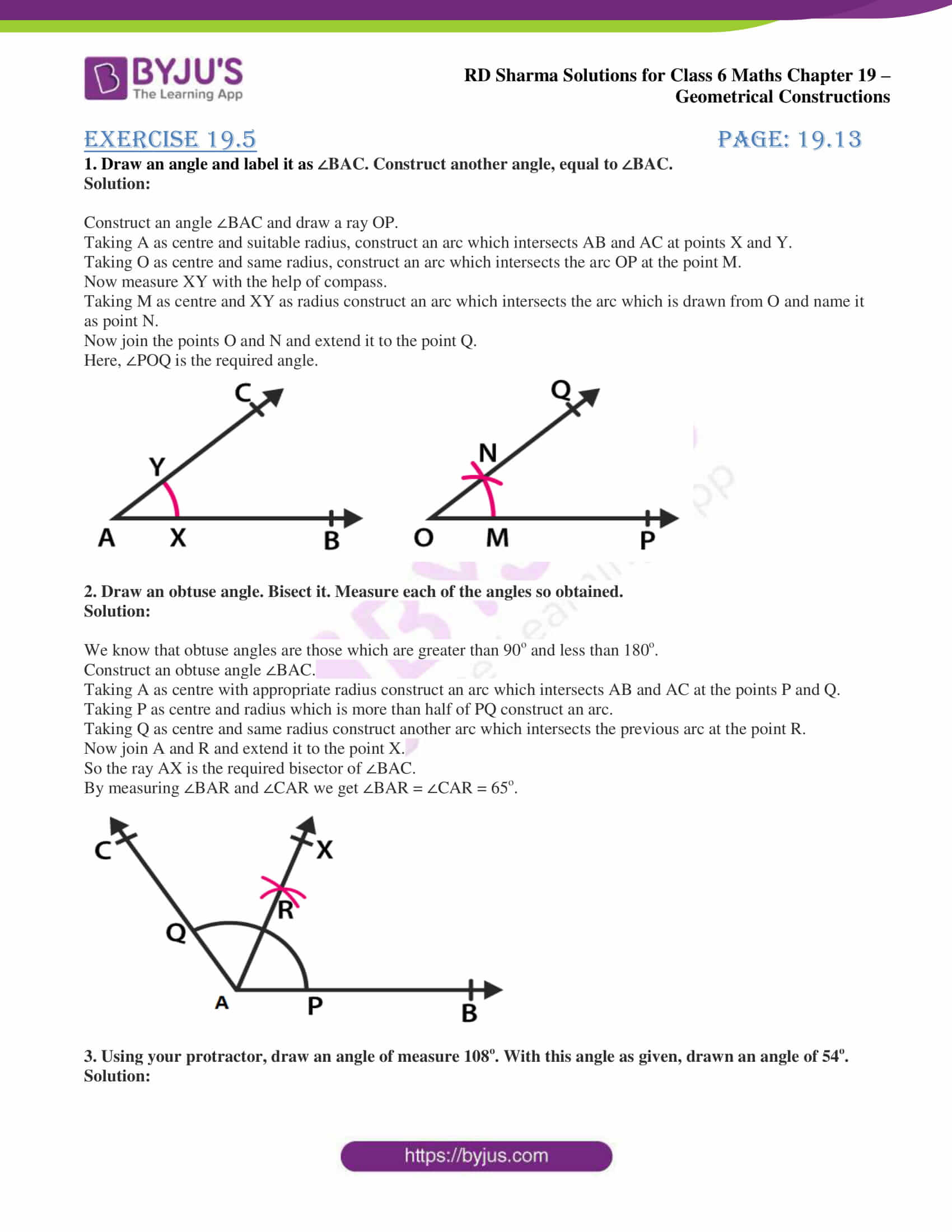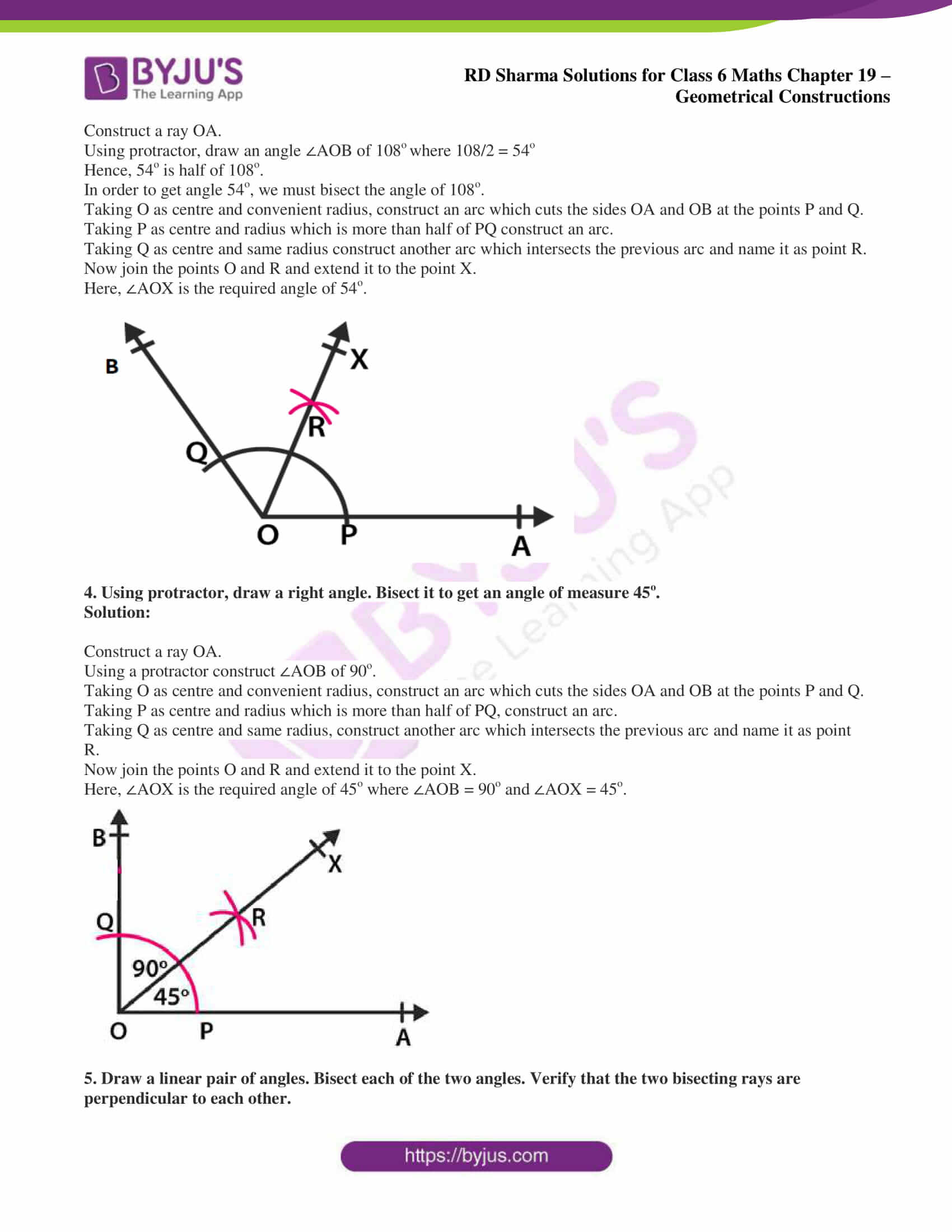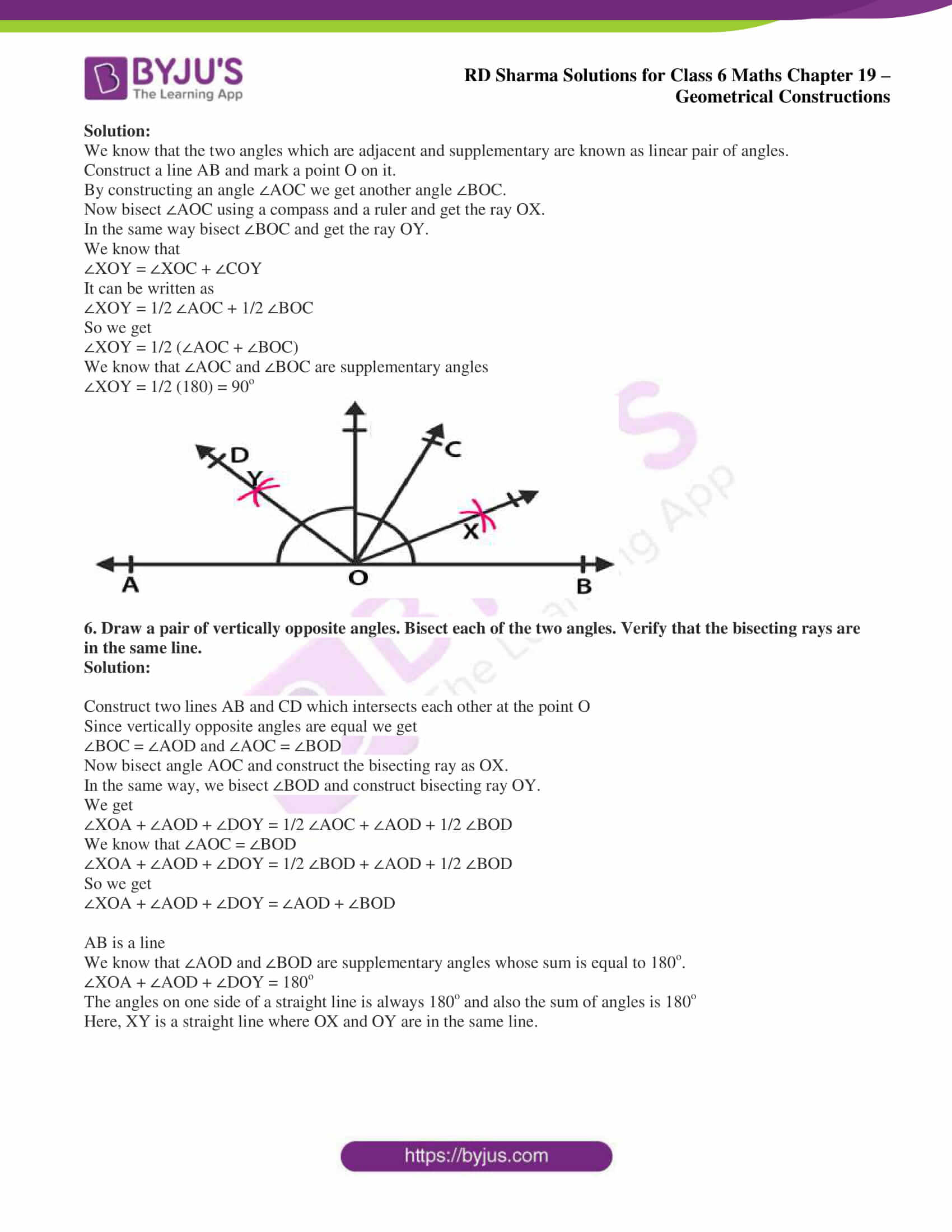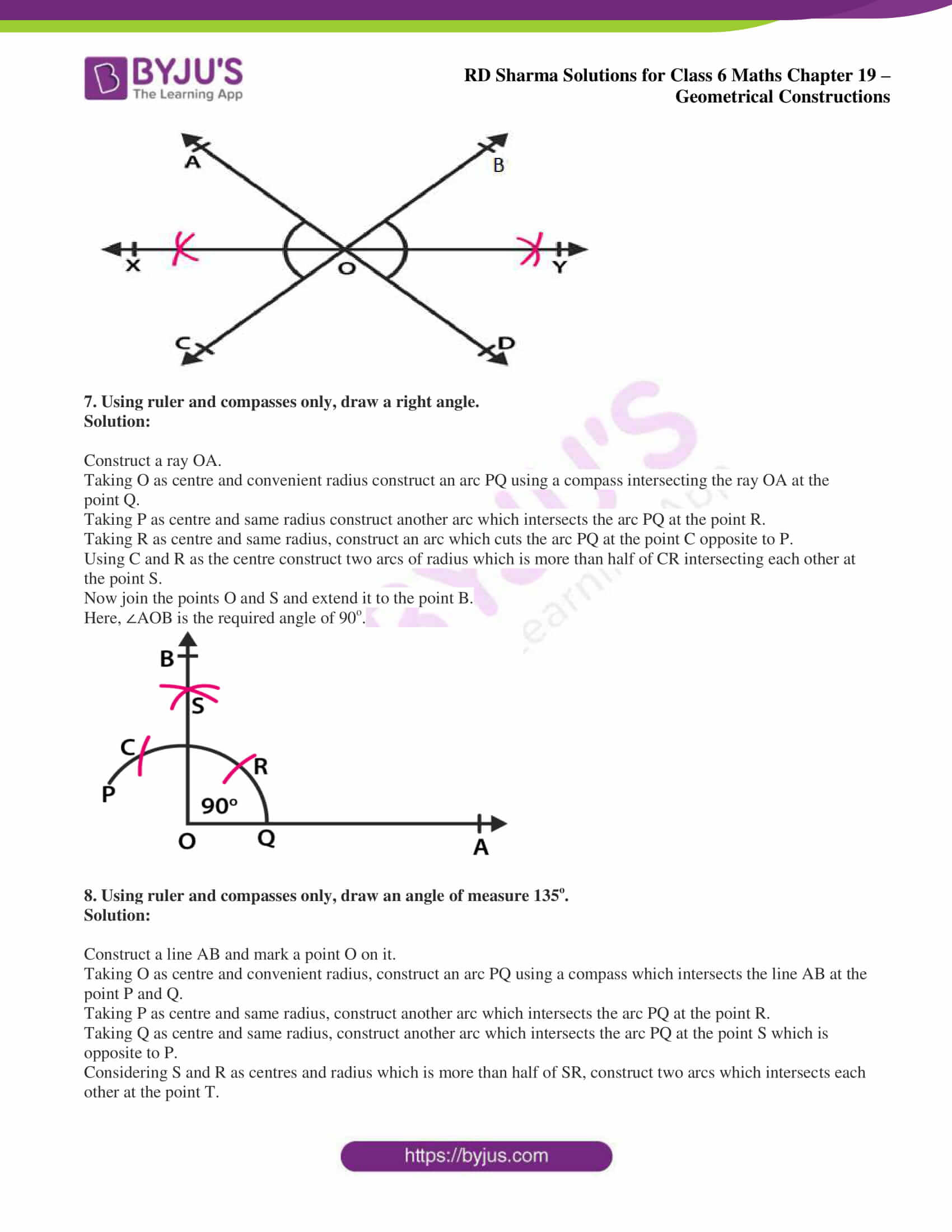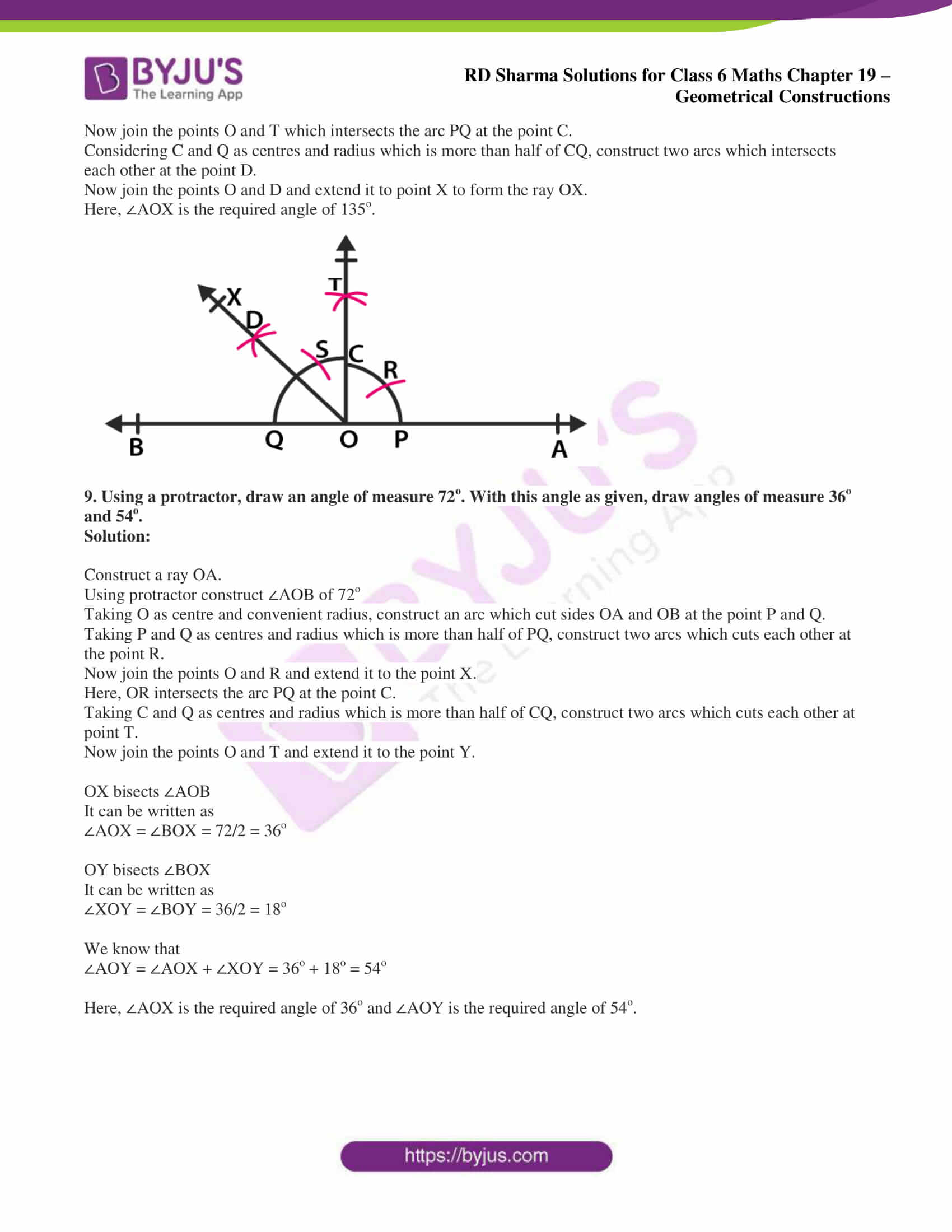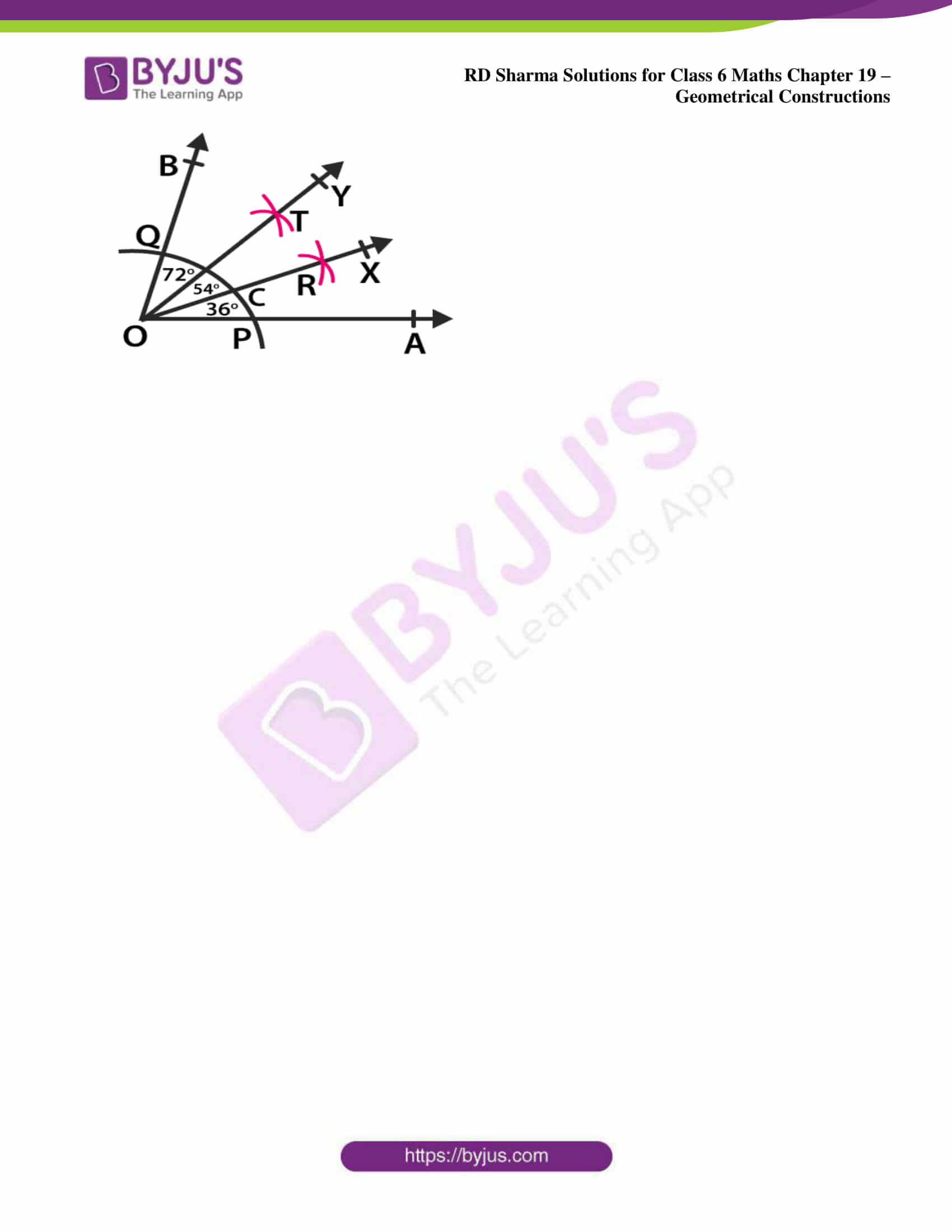### Exercise 19.5 page: 19.13

1. Draw an angle and label it as ∠BAC. Construct another angle, equal to ∠BAC.

Solution:

Construct an angle ∠BAC and draw a ray OP.

Taking A as centre and suitable radius, construct an arc which intersects AB and AC at points X and Y.

Taking O as centre and same radius, construct an arc which intersects the arc OP at the point M.

Now measure XY with the help of compass.

Taking M as centre and XY as radius construct an arc which intersects the arc which is drawn from O and name it as point N.

Now join the points O and N and extend it to the point Q.

Here, ∠POQ is the required angle.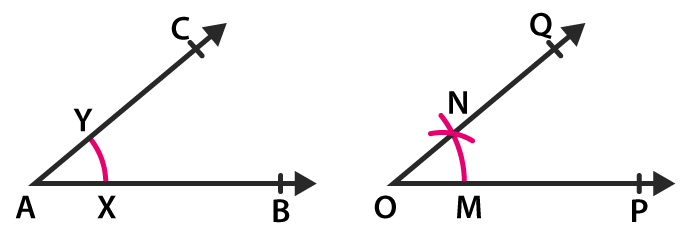2. Draw an obtuse angle. Bisect it. Measure each of the angles so obtained.

Solution:

We know that obtuse angles are those which are greater than 90o and less than 180o.

Construct an obtuse angle ∠BAC.

Taking A as centre with appropriate radius construct an arc which intersects AB and AC at the points P and Q.

Taking P as centre and radius which is more than half of PQ construct an arc.

Taking Q as centre and same radius construct another arc which intersects the previous arc at the point R.

Now join A and R and extend it to the point X.

So the ray AX is the required bisector of ∠BAC.

By measuring ∠BAR and ∠CAR we get ∠BAR = ∠CAR = 65o.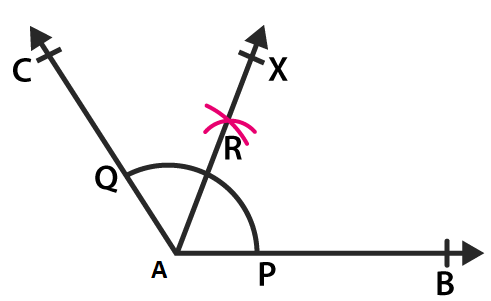3. Using your protractor, draw an angle of measure 108o. With this angle as given, drawn an angle of 54o.

Solution:

Construct a ray OA.

Using protractor, draw an angle ∠AOB of 108o where 108/2 = 54o

Hence, 54o is half of 108o.

In order to get angle 54o, we must bisect the angle of 108o.

Taking O as centre and convenient radius, construct an arc which cuts the sides OA and OB at the points P and Q.

Taking P as centre and radius which is more than half of PQ construct an arc.

Taking Q as centre and same radius construct another arc which intersects the previous arc and name it as point R.

Now join the points O and R and extend it to the point X.

Here, ∠AOX is the required angle of 54o.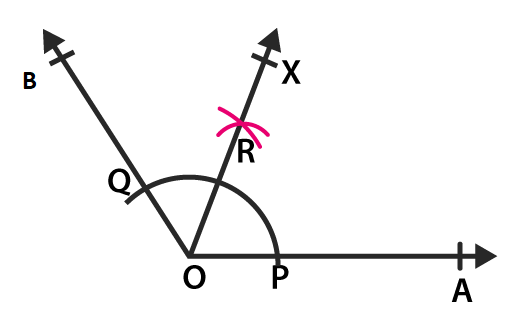4. Using protractor, draw a right angle. Bisect it to get an angle of measure 45o.

Solution:

Construct a ray OA.

Using a protractor construct ∠AOB of 90o.

Taking O as centre and convenient radius, construct an arc which cuts the sides OA and OB at the points P and Q.

Taking P as centre and radius which is more than half of PQ, construct an arc.

Taking Q as centre and same radius, construct another arc which intersects the previous arc and name it as point R.

Now join the points O and R and extend it to the point X.

Here, ∠AOX is the required angle of 45o where ∠AOB = 90o and ∠AOX = 45o.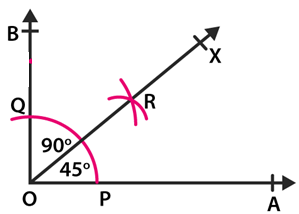5. Draw a linear pair of angles. Bisect each of the two angles. Verify that the two bisecting rays are perpendicular to each other.

Solution:

We know that the two angles which are adjacent and supplementary are known as linear pair of angles.

Construct a line AB and mark a point O on it.

By constructing an angle ∠AOC we get another angle ∠BOC.

Now bisect ∠AOC using a compass and a ruler and get the ray OX.

In the same way bisect ∠BOC and get the ray OY.

We know that

∠XOY = ∠XOC + ∠COY

It can be written as

∠XOY = 1/2 ∠AOC + 1/2 ∠BOC

So we get

∠XOY = 1/2 (∠AOC + ∠BOC)

We know that ∠AOC and ∠BOC are supplementary angles

∠XOY = 1/2 (180) = 90o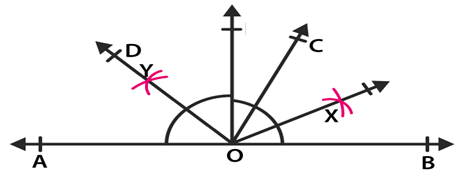6. Draw a pair of vertically opposite angles. Bisect each of the two angles. Verify that the bisecting rays are in the same line.

Solution:

Construct two lines AB and CD which intersects each other at the point O

Since vertically opposite angles are equal we get

∠BOC = ∠AOD and ∠AOC = ∠BOD

Now bisect angle AOC and construct the bisecting ray as OX.

In the same way, we bisect ∠BOD and construct bisecting ray OY.

We get

∠XOA + ∠AOD + ∠DOY = 1/2 ∠AOC + ∠AOD + 1/2 ∠BOD

We know that ∠AOC = ∠BOD

∠XOA + ∠AOD + ∠DOY = 1/2 ∠BOD + ∠AOD + 1/2 ∠BOD

So we get

∠XOA + ∠AOD + ∠DOY = ∠AOD + ∠BOD

AB is a line

We know that ∠AOD and ∠BOD are supplementary angles whose sum is equal to 180o.

∠XOA + ∠AOD + ∠DOY = 180o

The angles on one side of a straight line is always 180o and also the sum of angles is 180o

Here, XY is a straight line where OX and OY are in the same line.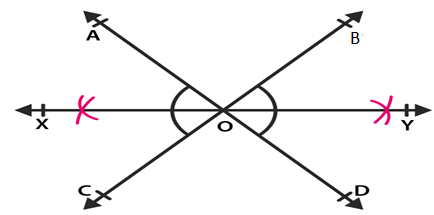7. Using ruler and compasses only, draw a right angle.

Solution:

Construct a ray OA.

Taking O as centre and convenient radius construct an arc PQ using a compass intersecting the ray OA at the point Q.

Taking P as centre and same radius construct another arc which intersects the arc PQ at the point R.

Taking R as centre and same radius, construct an arc which cuts the arc PQ at the point C opposite to P.

Using C and R as the centre construct two arcs of radius which is more than half of CR intersecting each other at the point S.

Now join the points O and S and extend it to the point B.

Here, ∠AOB is the required angle of 90o.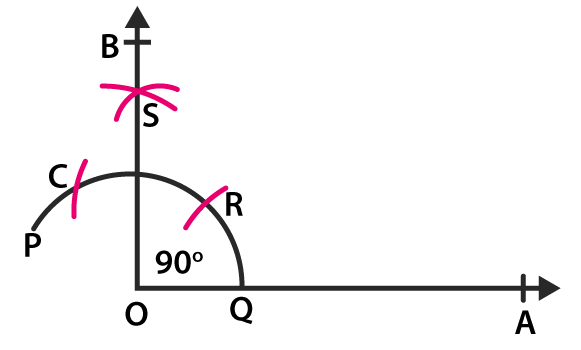8. Using ruler and compasses only, draw an angle of measure 135o.

Solution:

Construct a line AB and mark a point O on it.

Taking O as centre and convenient radius, construct an arc PQ using a compass which intersects the line AB at the point P and Q.

Taking P as centre and same radius, construct another arc which intersects the arc PQ at the point R.

Taking Q as centre and same radius, construct another arc which intersects the arc PQ at the point S which is opposite to P.

Considering S and R as centres and radius which is more than half of SR, construct two arcs which intersects each other at the point T.

Now join the points O and T which intersects the arc PQ at the point C.

Considering C and Q as centres and radius which is more than half of CQ, construct two arcs which intersects each other at the point D.

Now join the points O and D and extend it to point X to form the ray OX.

Here, ∠AOX is the required angle of 135o.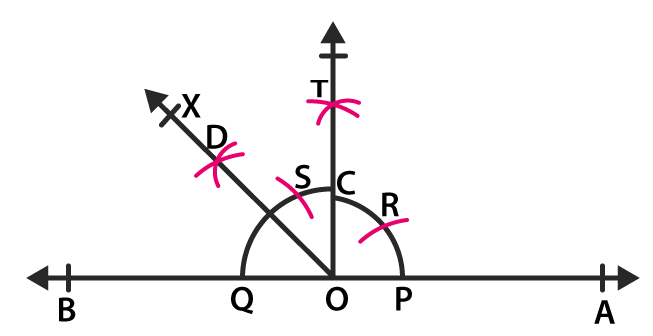9. Using a protractor, draw an angle of measure 72o. With this angle as given, draw angles of measure 36o and 54o.

Solution:

Construct a ray OA.

Using protractor construct ∠AOB of 72o

Taking O as centre and convenient radius, construct an arc which cut sides OA and OB at the point P and Q.

Taking P and Q as centres and radius which is more than half of PQ, construct two arcs which cuts each other at the point R.

Now join the points O and R and extend it to the point X.

Here, OR intersects the arc PQ at the point C.

Taking C and Q as centres and radius which is more than half of CQ, construct two arcs which cuts each other at point T.

Now join the points O and T and extend it to the point Y.

OX bisects ∠AOB

It can be written as

∠AOX = ∠BOX = 72/2 = 36o

OY bisects ∠BOX

It can be written as

∠XOY = ∠BOY = 36/2 = 18o

We know that

∠AOY = ∠AOX + ∠XOY = 36o + 18o = 54o

Here, ∠AOX is the required angle of 36o and ∠AOY is the required angle of 54o.